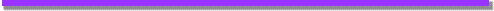Keyword Index

# Object: SPRect

Represents a rectangle, which may be represented using integer or real values

• bottom - Gets or sets the bottom coordinate of the rectangle
• center - Returns a point representing the center of the rectangle. If the rectangle contains only integer values, the point will be rounded to the nearest integer position, otherwise a real point is returned
• height - Gets or sets the height of the rectangle, i.e. sets bottom = top + height
• inset - Inset (reduce size) of the rectangle by the given amount, or increase it if negative values are given
• isReal - Returns true if either of the coordinates contains a real value, false if both are integers
• left - Gets or sets the left coordinate of the rectangle
• right - Gets or sets the right coordinate of the rectangle
• top - Gets or sets the top coordinate of the rectangle
• width - Gets or sets the width of the rectangle, i.e. sets right = left + width
•Property: bottom

Gets or sets the bottom coordinate of the rectangleMethod: center

Returns a point representing the center of the rectangle. If the rectangle contains only integer values, the point will be rounded to the nearest integer position, otherwise a real point is returnedProperty: height

Gets or sets the height of the rectangle, i.e. sets bottom = top + heightMethod: inset

Inset (reduce size) of the rectangle by the given amount, or increase it if negative values are given

void inset(x);

void inset(x, y);

Parameters
Name Expected Type Description
y int amount to inset top and bottom. If negative, the dimensions are expanded. If y is not specified, it is the same as x
x int amount to inset left and right. If negative, the dimensions are expandedProperty: isReal

Returns true if either of the coordinates contains a real value, false if both are integersProperty: left

Gets or sets the left coordinate of the rectangleProperty: right

Gets or sets the right coordinate of the rectangleProperty: top

Gets or sets the top coordinate of the rectangleProperty: width

Gets or sets the width of the rectangle, i.e. sets right = left + width